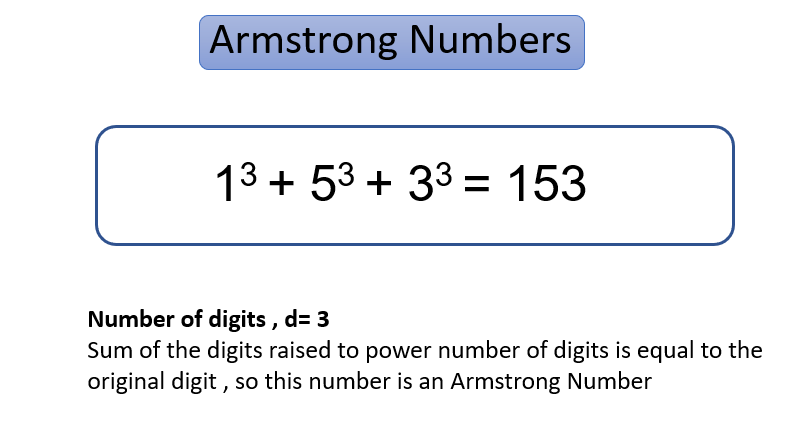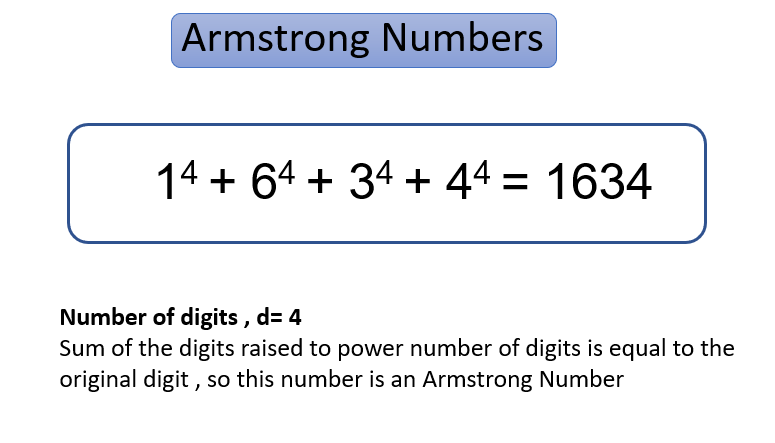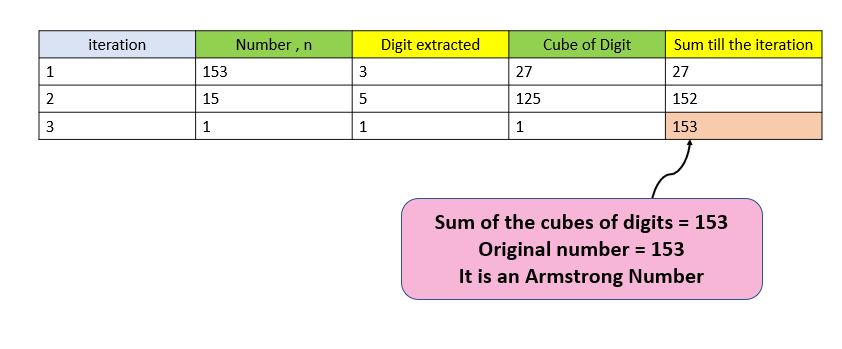# Check if a number is Armstrong Number or not

Problem Statement: Given a number, check if it is Armstrong Number or Not.

Examples:

```Example 1:
Input:153
Output: Yes, it is an Armstrong Number
Explanation: 1^3 + 5^3 + 3^3 = 153

Input:170
Output: No, it is not an Armstrong Number
Explanation: 1^3 + 7^3 + 0^3 != 170```

What are Armstrong Numbers?

Armstrong Numbers are the numbers having the sum of digits raised to power no. of digits is equal to a given number. Examples- 0, 1, 153, 370, 371, 407, and 1634 are some of the Armstrong Numbers.### Solution

Approach:

Let us check one 3-digit Armstrong Number

n=153

sum=0

No. of digits = 3 so we need to cube every digit.

In the First iteration, extract digit 3 from 153 and cube it which becomes 27, add it to sum= 0+27=27 which becomes 27 now

In Second iteration , extract digit 5 from 15 and cube it which becomes 125 , add it to sum = 27 +125 = 152 which becomes 152 now

In Third iteration , extract digit 1 from 1 and cube it which becomes 1 , add it to sum = 152 + 1 = 153 which becomes 153 now.

The original Number was 153 and the sum of cubes = 153.

This means it is an Armstrong Number.Code:

## C++ Code

``````#include <bits/stdc++.h>
using namespace std;
bool ArmstrongNumber(int n)
{
int originalno = n;
int count = 0;
int temp = n;
while (temp != 0)
{
count++;
temp = temp / 10;
}
int sumofpower = 0;
while (n != 0)
{
int digit = n % 10;
sumofpower += pow(digit,count);
n /= 10;
}
return (sumofpower == originalno);
}
int main()
{
int n1 = 153;
if (ArmstrongNumber(n1))
{
cout << "Yes, it is an Armstrong Number\n";
}
else
{
cout << "No, it is not an Armstrong Number\n";
}
return 0;
}
``````

Output:

Yes, it is an Armstrong Number

Time Complexity: O(n) where n is the number of digits since we need to traverse every digit and add digits raised to power no. of digits to sum.

Space Complexity: O(1) since no extra space is required

## Java Code

``````import java.util.*;
class TUF{
static boolean ArmstrongNumber(int n)
{
int originalno = n;
int count = 0;
int temp = n;
while (temp != 0)
{
count++;
temp = temp / 10;
}
int sumofpower = 0;
while (n != 0)
{
int digit = n % 10;
sumofpower += Math.pow(digit,count);
n /= 10;
}
return (sumofpower == originalno);
}
public static void main(String args[])
{
int n1 = 153;
if (ArmstrongNumber(n1))
{
System.out.println("Yes, it is an Armstrong Number\n");
}
else
{
System.out.println("No, it is not an Armstrong Number\n");
}

}
}``````

Output:

Yes, it is an Armstrong Number

Time Complexity: O(n) where n is the number of digits since we need to traverse every digit and add digits raised to power no. of digits to sum.

Space Complexity: O(1) since no extra space is required

Special thanks to Gurmeet Singh for contributing to this article on takeUforward. If you also wish to share your knowledge with the takeUforward fam, please check out this article